# RD Sharma Solutions For Class 12 Maths Exercise 19.8 Chapter 19 Indefinite Integrals

RD Sharma Solutions for Class 12 Maths Exercise 19.8 Chapter 19 Indefinite Integrals is provided here. Problems pertaining to examining the integrals of the form first-order derivative in the numerator in the integrand is the exact differential of the denominator, are dealt with in this exercise. The solutions to this chapter are formulated by our expert tutors, to boost the confidence levels of students in understanding the concepts and methods to solve problems in a shorter period.

RD Sharma Class 12 Maths Solutions are of immense help to students aiming to secure a good academic score in the board exams. To know more about this topic, students can download the RD Sharma Solutions for Class 12 Maths Chapter 19 Exercise 19.8 from the below-mentioned links.

## Download the PDF of RD Sharma Solutions For Class 12 Chapter 19 – Indefinite Integrals Exercise 19.8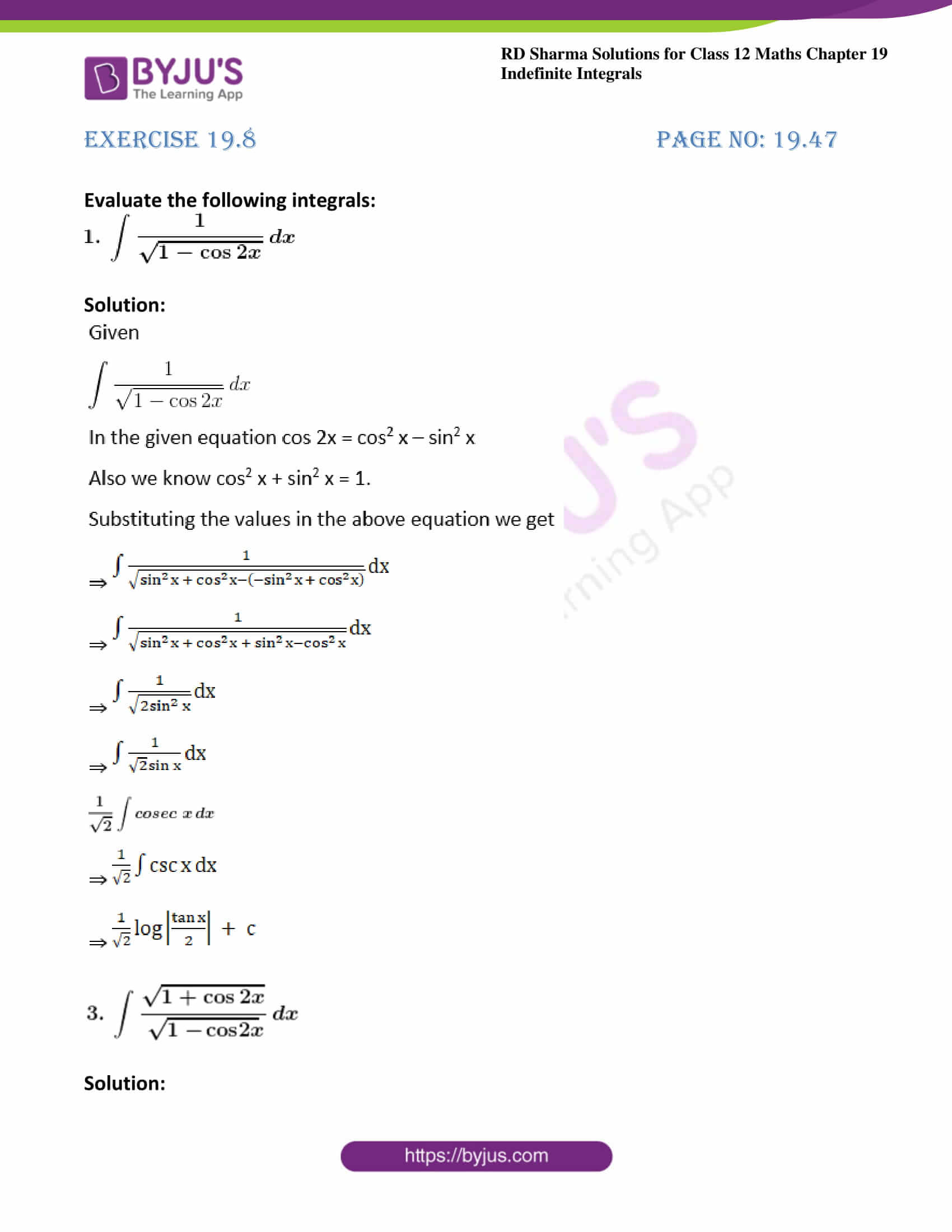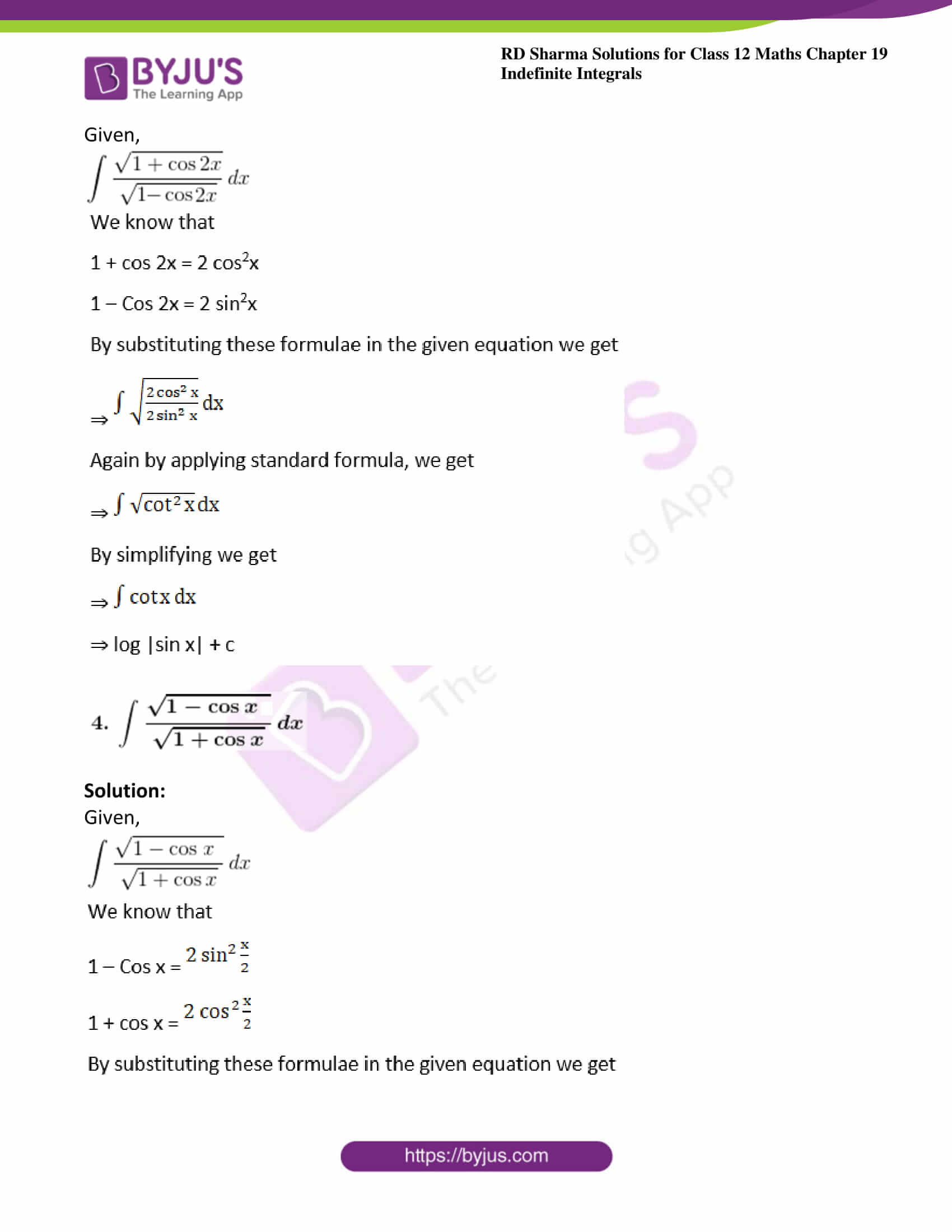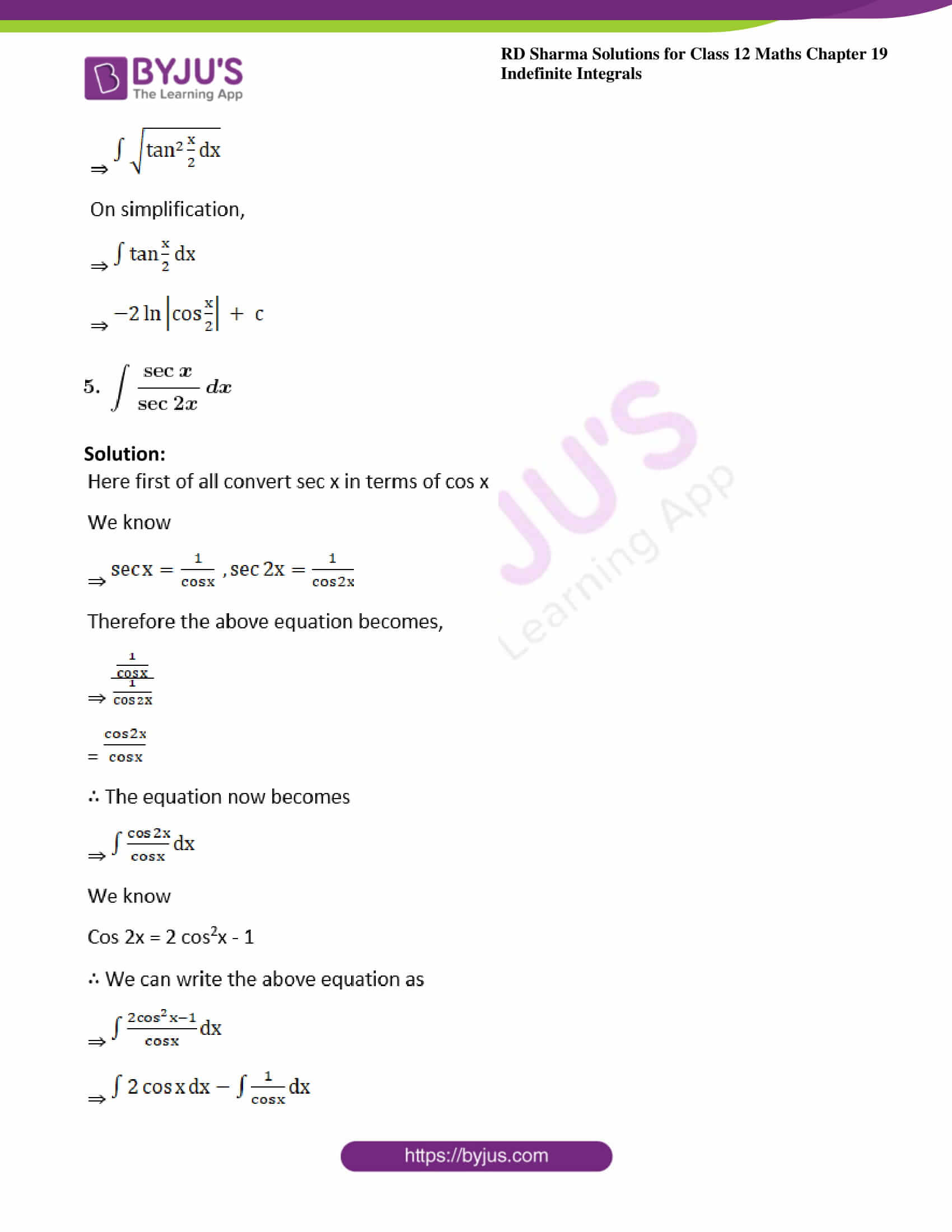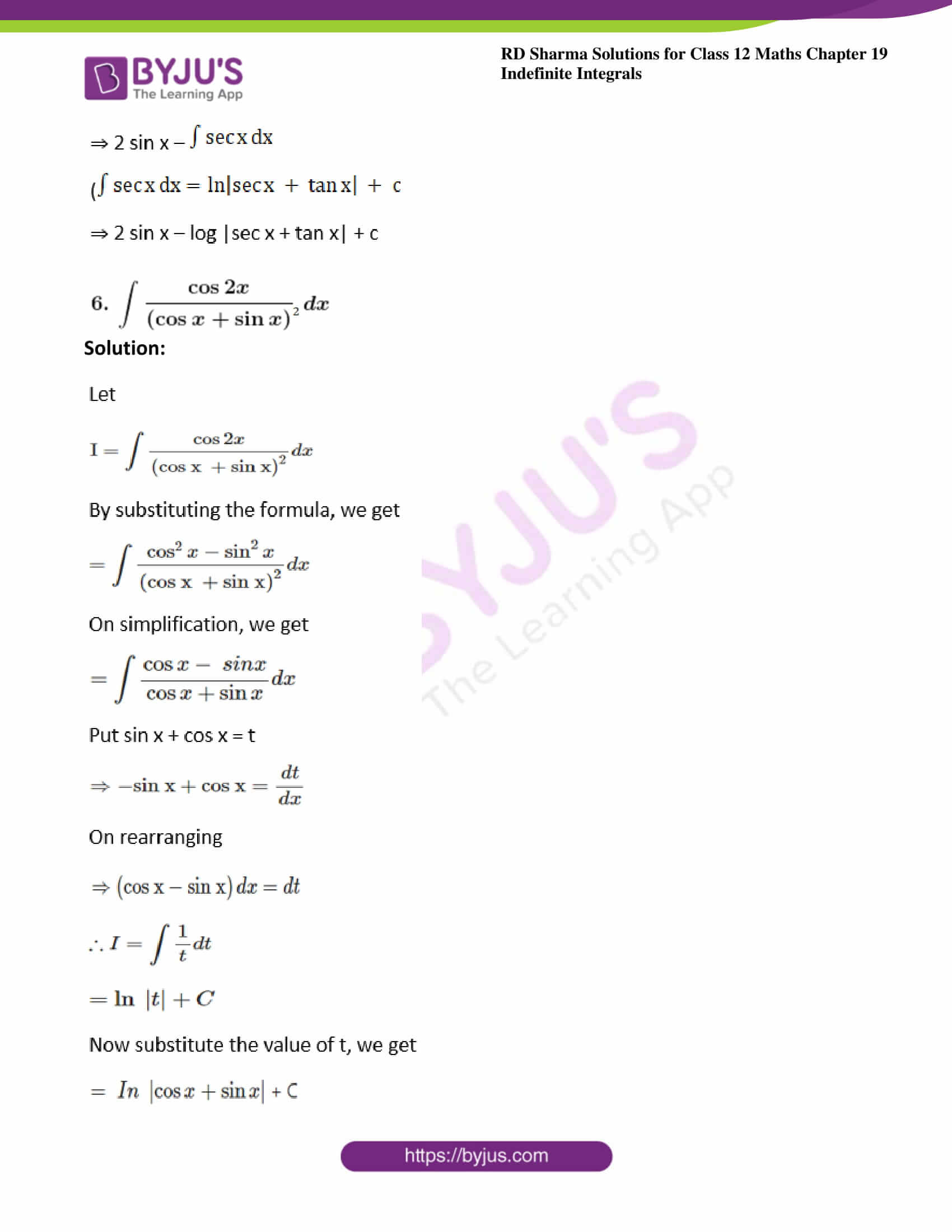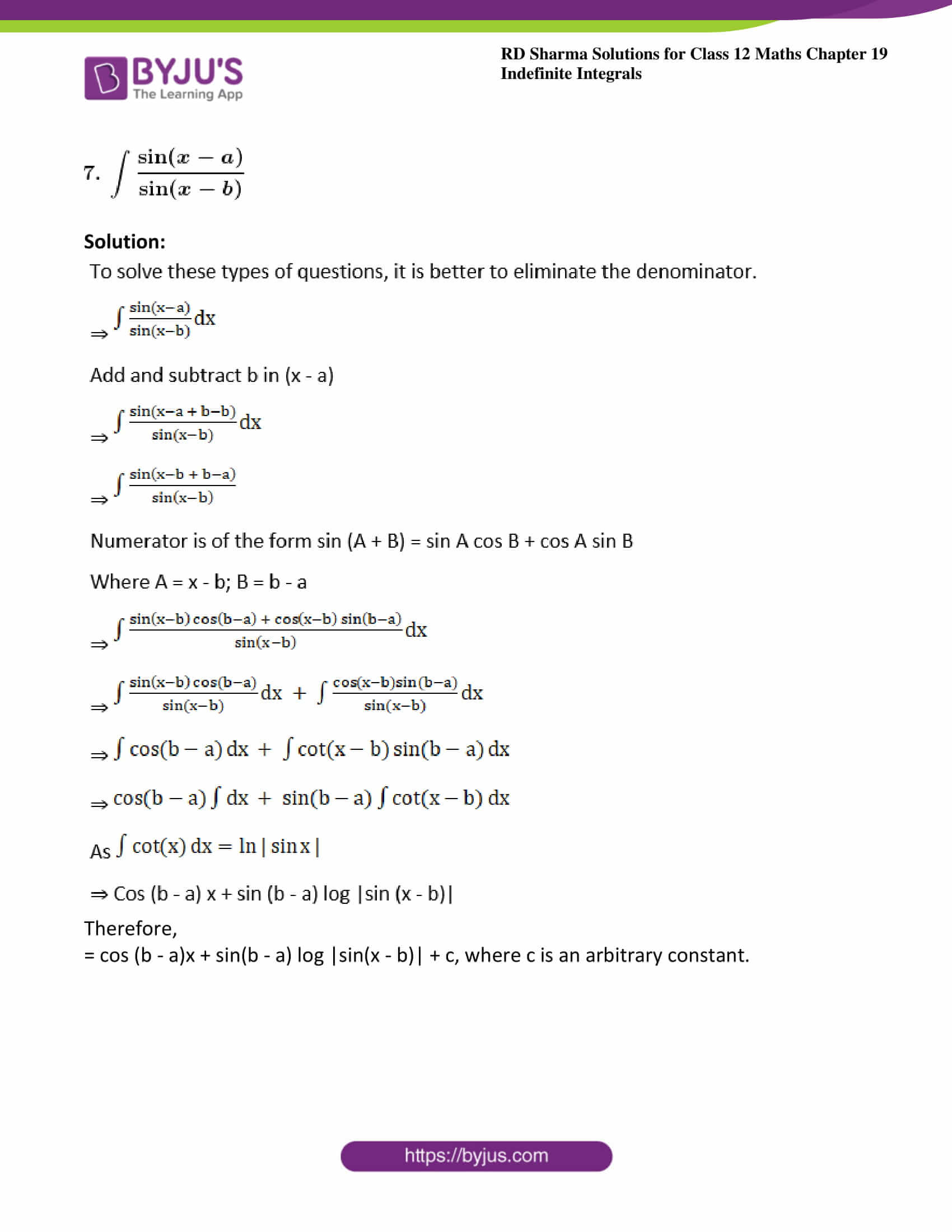### Exercise 19.8 Page No: 19.47

Evaluate the following integrals: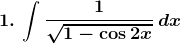Solution: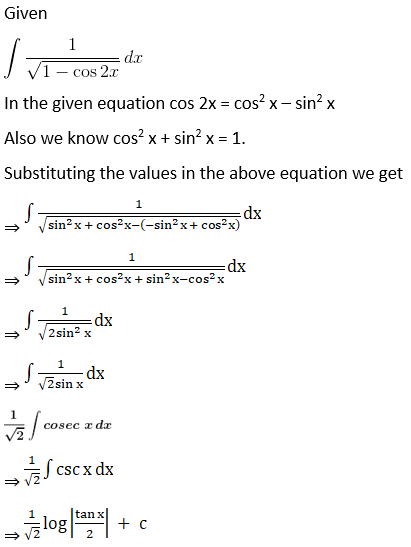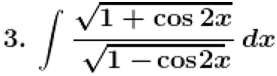Solution:

Given,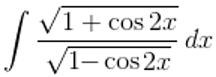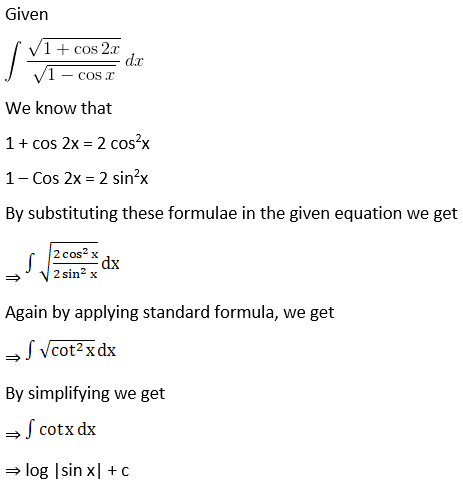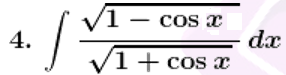Solution:

Given,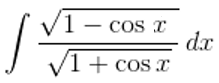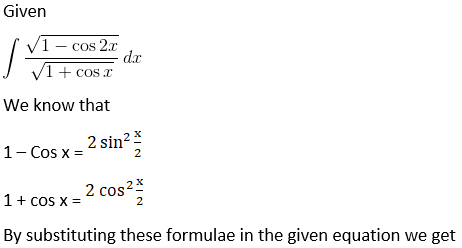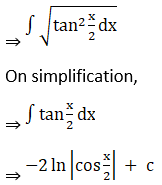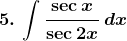Solution: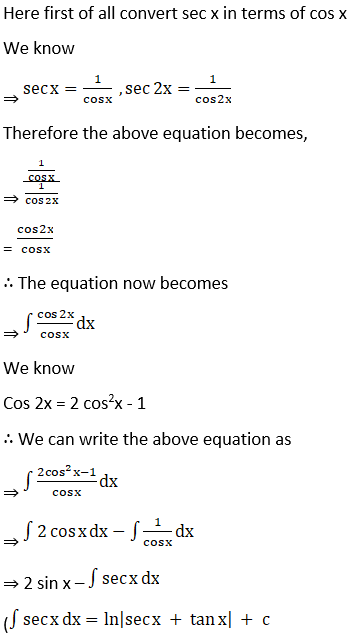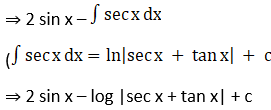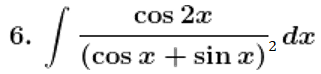Solution: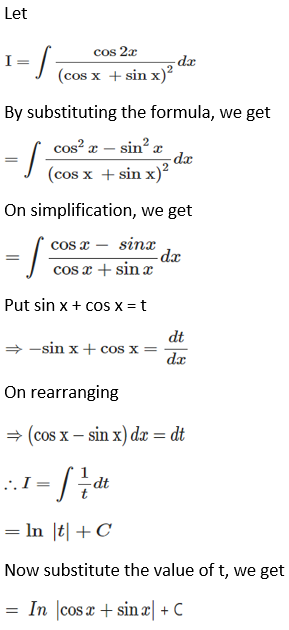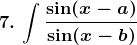Solution: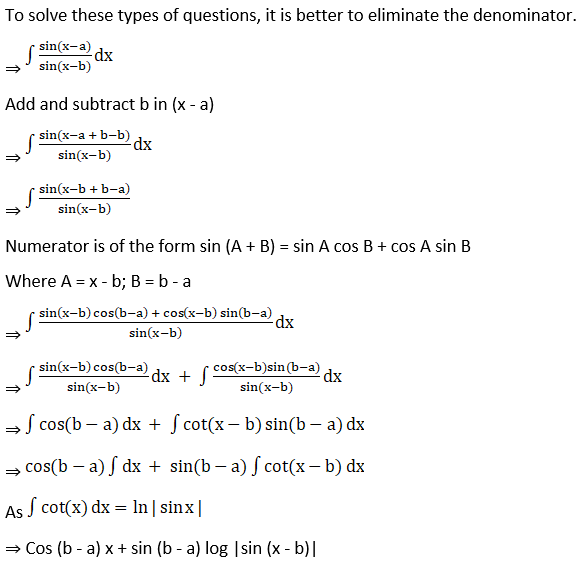Therefore,

= cos (b – a)x + sin(b – a) log |sin(x – b)| + c, where c is an arbitrary constant.

### Access other exercises of RD Sharma Solutions For Class 12 Chapter 19 – Indefinite Integrals

Exercise 19.1 Solutions

Exercise 19.2 Solutions

Exercise 19.3 Solutions

Exercise 19.4 Solutions

Exercise 19.5 Solutions

Exercise 19.6 Solutions

Exercise 19.7 Solutions

Exercise 19.9 Solutions

Exercise 19.10 Solutions

Exercise 19.11 Solutions

Exercise 19.12 Solutions

Exercise 19.13 Solutions

Exercise 19.14 Solutions

Exercise 19.15 Solutions

Exercise 19.16 Solutions

Exercise 19.17 Solutions

Exercise 19.18 Solutions

Exercise 19.19 Solutions

Exercise 19.20 Solutions

Exercise 19.21 Solutions

Exercise 19.22 Solutions

Exercise 19.23 Solutions

Exercise 19.24 Solutions

Exercise 19.25 Solutions

Exercise 19.26 Solutions

Exercise 19.27 Solutions

Exercise 19.28 Solutions

Exercise 19.29 Solutions

Exercise 19.30 Solutions

Exercise 19.31 Solutions

Exercise 19.32 Solutions